Practice the problems of Math in Focus Grade 4 Workbook Answer Key Chapter 6 Practice 1 Adding Fractions to score better marks in the exam.

Find the equivalent fraction. Complete the model. Then add.

Example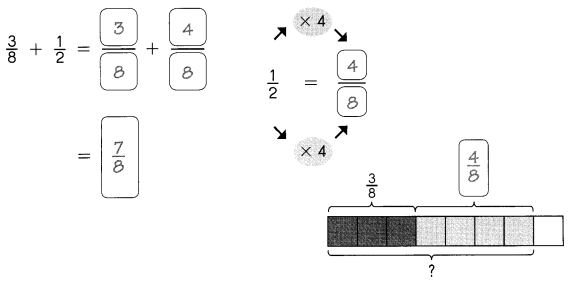Question 1.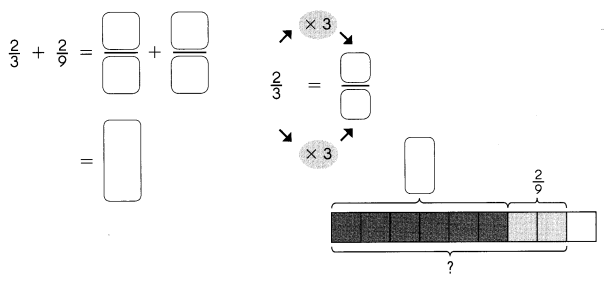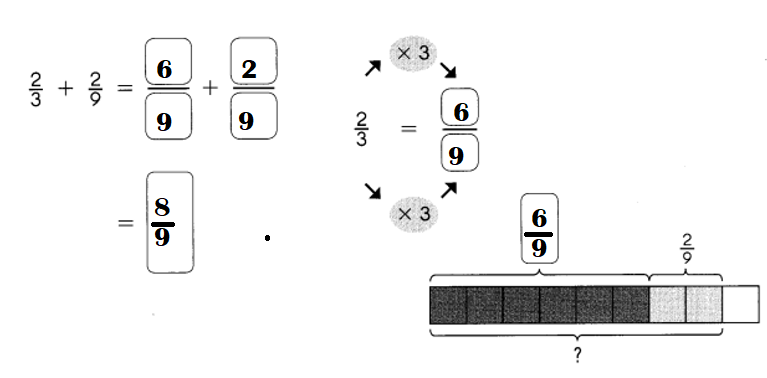Explanation:
$$\frac{2}{3}$$ + $$\frac{2}{9}$$
= (6 + 2) ÷ 9
= 8 ÷ 9 or + $$\frac{8}{9}$$

Question 2.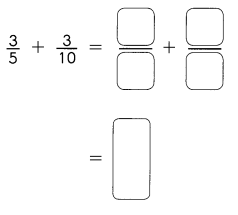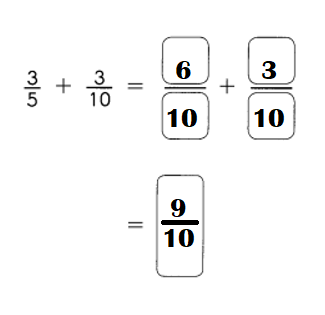Explanation:
$$\frac{3}{5}$$ + $$\frac{3}{10}$$
= (6 + 3) ÷ 10
= 9 ÷ 10 or $$\frac{9}{10}$$

Question 3.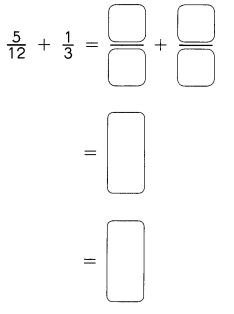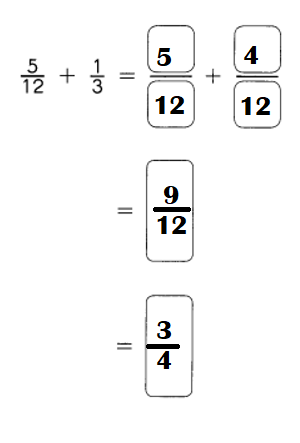Explanation:
$$\frac{5}{12}$$ + $$\frac{1}{3}$$
= (5 + 4) ÷ 12
= 9 ÷ 12
= 3 ÷ 4 or $$\frac{3}{4}$$

Question 4.
Find the sum of $$\frac{1}{6}$$ and $$\frac{1}{12}$$.
$$\frac{1}{6}$$ + $$\frac{1}{12}$$  = $$\frac{1}{4}$$

Explanation:
Sum:
$$\frac{1}{6}$$ + $$\frac{1}{12}$$
= (2 + 1) ÷ 12
= 3 ÷ 12
= $$\frac{1}{4}$$

Question 5.
Add $$\frac{1}{4}$$ to the answer in Exercise 4.
$$\frac{1}{4}$$ + $$\frac{1}{4}$$  = 1 ÷ 2 or $$\frac{1}{2}$$

Explanation:
Sum:
$$\frac{1}{4}$$ + $$\frac{1}{4}$$
= (1 + 1) ÷ 4
= 2 ÷ 4
= 1 ÷ 2 or $$\frac{1}{2}$$

Question 6.
What is the sum of $$\frac{1}{8}$$, $$\frac{1}{4}$$ and $$\frac{3}{8}$$?
$$\frac{1}{8}$$ + $$\frac{1}{4}$$ + $$\frac{3}{8}$$ = 3 ÷ 4 or $$\frac{3}{4}$$

Explanation:
Sum:
$$\frac{1}{8}$$ + $$\frac{1}{4}$$ + $$\frac{3}{8}$$
= (1 + 2) ÷ 8 + $$\frac{3}{8}$$
= $$\frac{3}{8}$$ + $$\frac{3}{8}$$
= (3 + 3) ÷ 8
= 6 ÷ 8
= 3 ÷ 4 or $$\frac{3}{4}$$

Question 7.
Add $$\frac{1}{3}$$, $$\frac{3}{12}$$ and $$\frac{5}{12}$$
$$\frac{1}{3}$$ + $$\frac{3}{12}$$ + $$\frac{5}{12}$$ = 1.
$$\frac{1}{3}$$ + $$\frac{3}{12}$$ + $$\frac{5}{12}$$
= (4 + 3) ÷ 12 + $$\frac{5}{12}$$
= $$\frac{7}{12}$$ + $$\frac{5}{12}$$